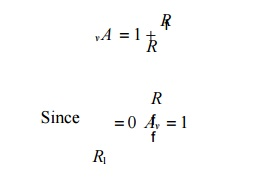Home | | Linear Integrated Circuits | Voltage follower

# Voltage follower

1. Voltage to Current Converter with floating loads (V/I): 2. Voltage – to current converter with Grounded load:

Voltage follower:

If R1=∞ and Rf=0 in the non inverting amplifier configuration .The amplifier act as a unity-gain amplifier or voltage follower.

That isThe circuit consist of an op-amp and a wire connecting the output voltage to the input ,i.e the output voltage is equal to the input voltage, both in magnitude and phase.V0=Vi

Since the output voltage of the circuit follows the input voltage, the circuit is called voltage follower. It offers very high input impedance of the order of MΩ and very low output impedance.

Therefore, this circuit draws negligible current from the source. Thus, the voltage follower can be used as a buffer between a high impedance source and a low impedance load for impedance matching applications.1. Voltage to Current Converter with floating loads (V/I):

1.        Voltage to current converter in which load resistor RL is floating (not connected to ground).

2.        Vin is applied to the non inverting input terminal, and the feedback voltage across R1 devices the inverting input terminal.

3.        This circuit is also called as a current – series negative feedback amplifier.

4. Because the feedback voltage across R1 (applied Non-inverting terminal) depends on the output current i0 and is in series with the input difference voltage VidFrom the fig input voltage Vin is converted into output current of Vin/R1 [Vin ->i0 ] . In other words, input volt appears across R1. If R1 is a precision resistor, the output current (i0 = Vin/R1 ) will be precisely fixed.

Applications:

1.        Low voltage ac and dc voltmeters

2.        Diode match finders

3.        LED

4.        Zener diode testers.

2. Voltage – to current converter with Grounded load:

This is the other type V – I converter, in which one terminal of the load is connected to ground.Analysis of the circuit:

The analysis of the circuit can be done by following 2 steps.

1.        To determine the voltage V1 at the non-inverting (+) terminals and

2.        To establish relationship between V1 and the load current IL .

Applying KCL at node V1 we can write that,

Study Material, Lecturing Notes, Assignment, Reference, Wiki description explanation, brief detail
Linear Integrated Ciruits : Application of Op-Amp : Voltage follower |